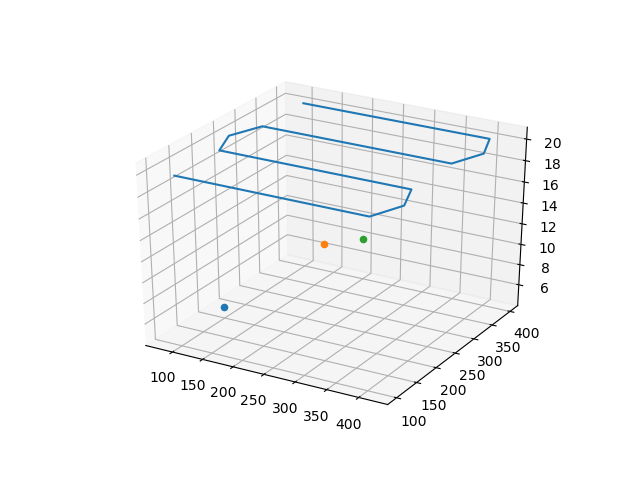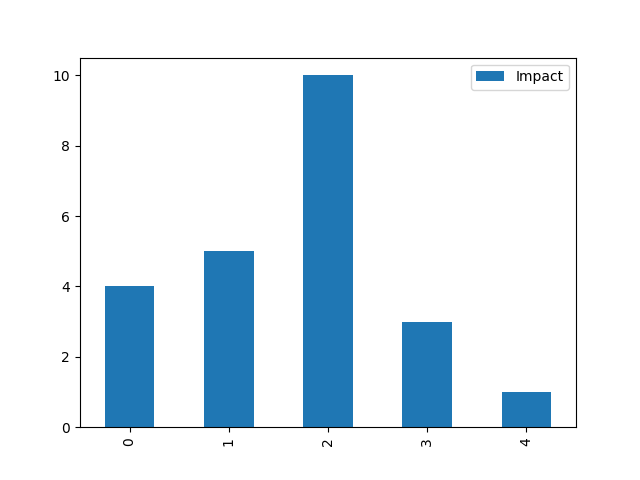# Graphics¶

The `chama.graphics` module provides methods to help visualize results.

## Signal graphics¶

Chama provides several functions to visualize signals described in the Simulation section (XYZ format only). Visualization is useful to verify that the signal was loaded/generated as expected, compare scenarios, and to better understand optimal sensor placement.

The convex hull of several scenarios can be generated as follows (Figure 3):

```>>> chama.graphics.signal_convexhull(signal, scenarios=['S1', 'S2', 'S3'], threshold=0.01)
```

The cross section of a single scenarios can be generated as follows (Figure 4):

```>>> chama.graphics.signal_xsection(signal, 'S1', threshold=0.01)
```

## Sensor graphics¶

The position of fixed and mobile sensors, described in the Sensor technology section, can be plotted. After grouping sensors in a dictionary, the locations can be plotted as follows (Figure 5):

```>>> chama.graphics.sensor_locations(sensors)
```Figure 5 Mobile and stationary sensor locations plot

After running a series of sensor placement optimizations with increasing sensor budget, a tradeoff curve can be generated using the objective value and fraction of detected scenarios. Figure 6 compares the expected time to detection and scenario coverage as the sensor budget increases.

## Scenario analysis¶

The impact of individual scenarios can also be analyzed for a single sensor placement using the optimization assessment. Figure 7 compares time to detection from several scenarios, given an optimal placement.

```>>> print(results['Assessment'])
Scenario Sensor  Impact
0       S1      A       4
1       S2      A       5
2       S3      B      10
3       S4      C       3
4       S5      A       1
>>> results['Assessment'].plot(kind='bar')
```Figure 7 Scenario impact values based on optimal placement